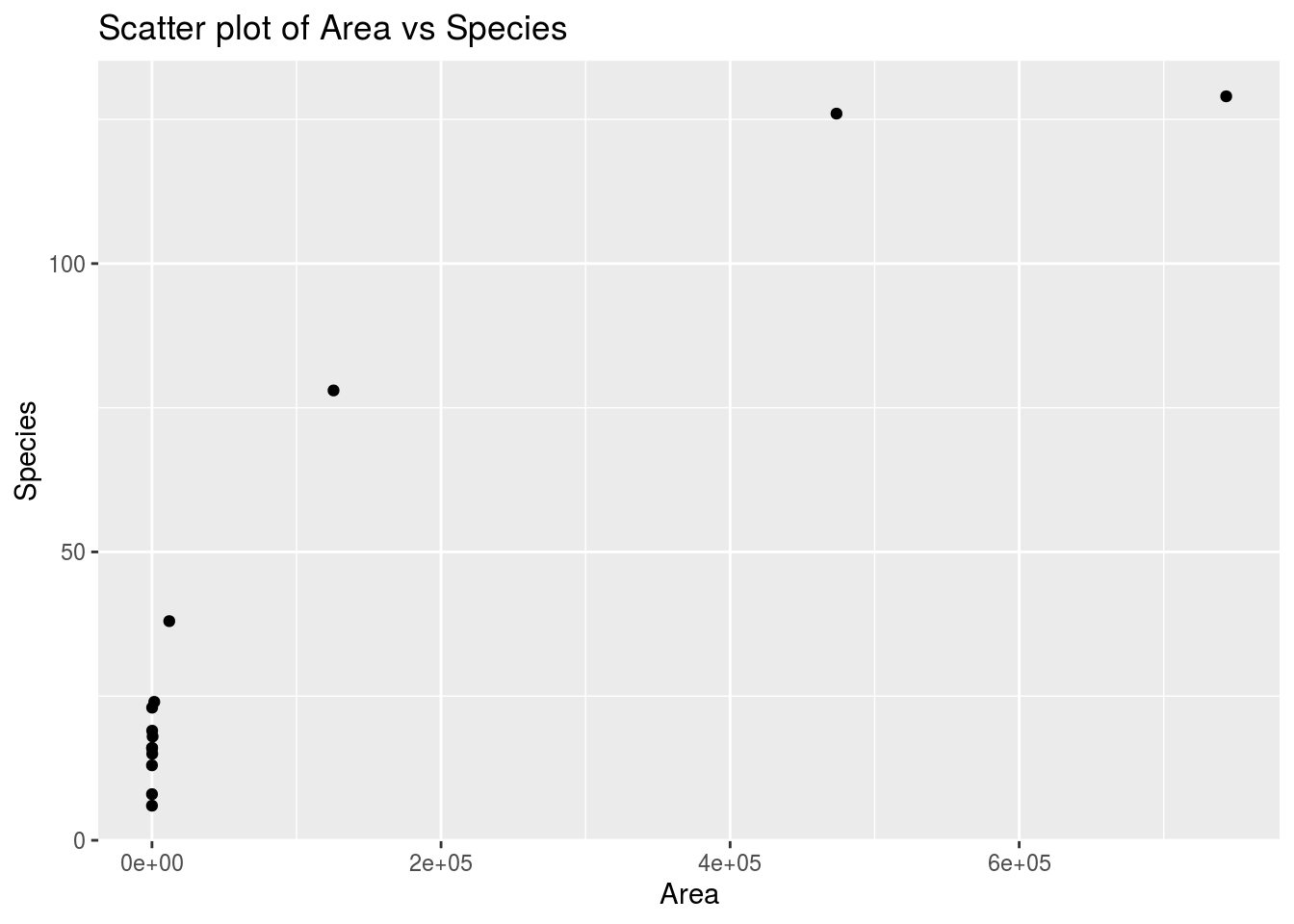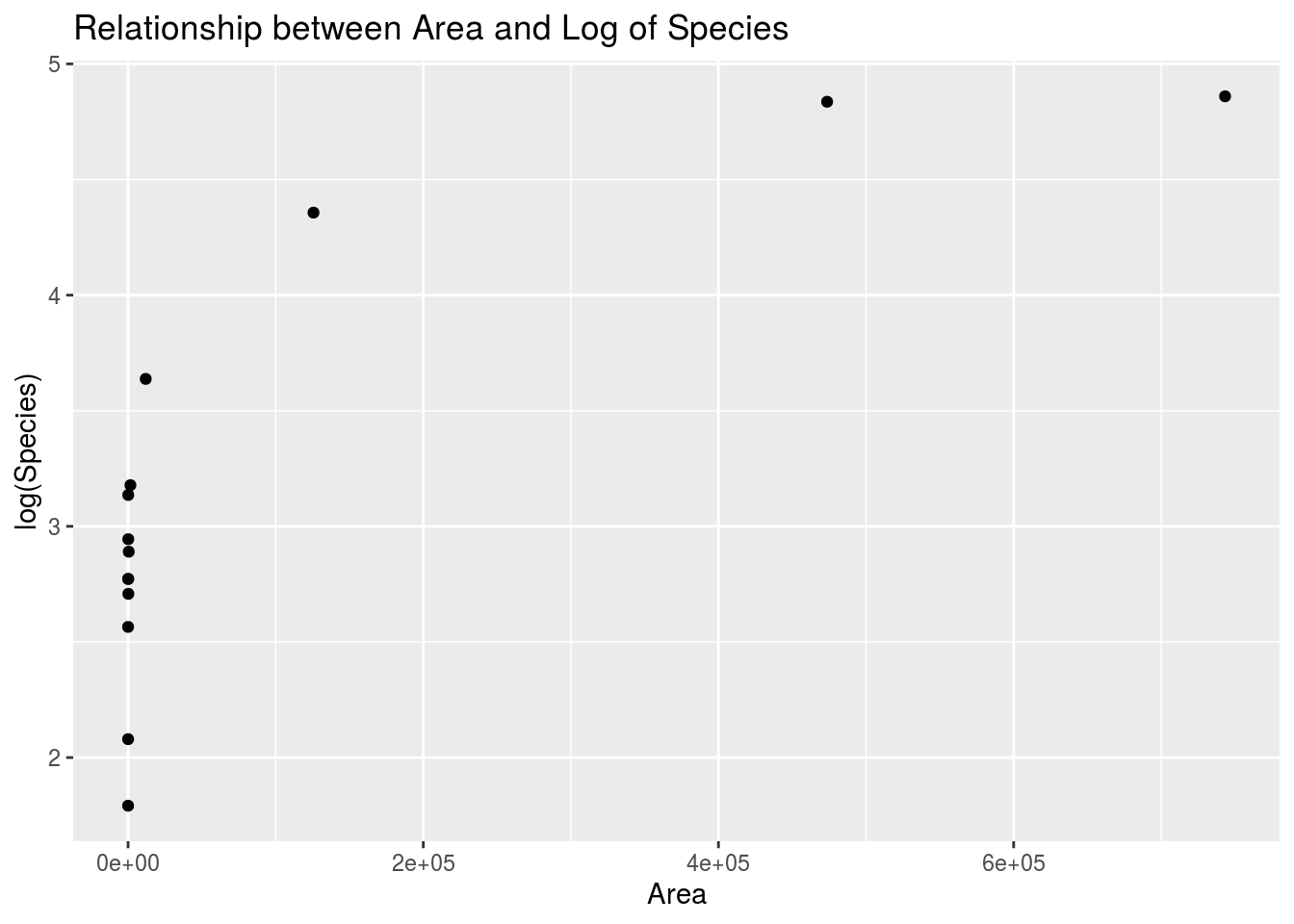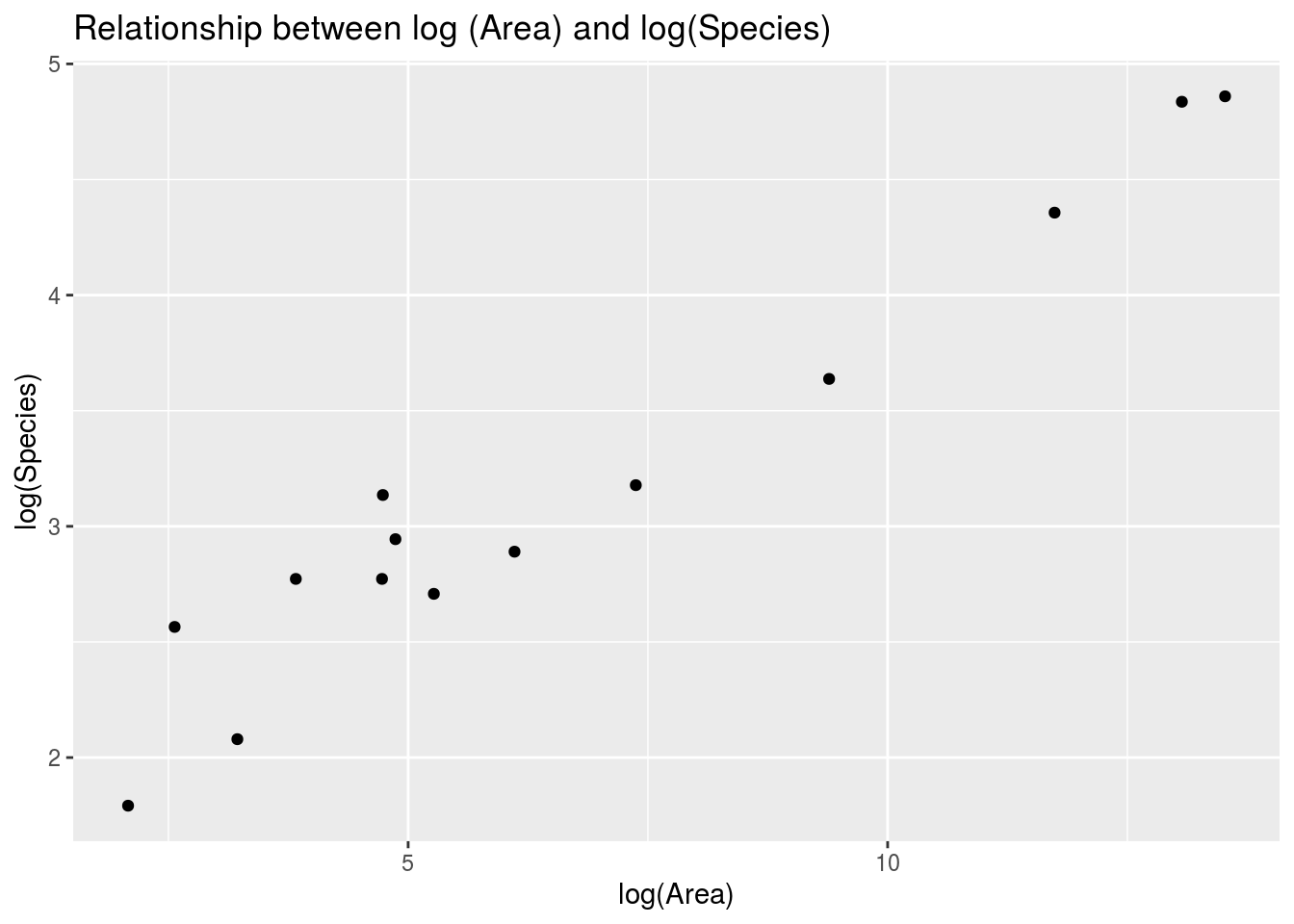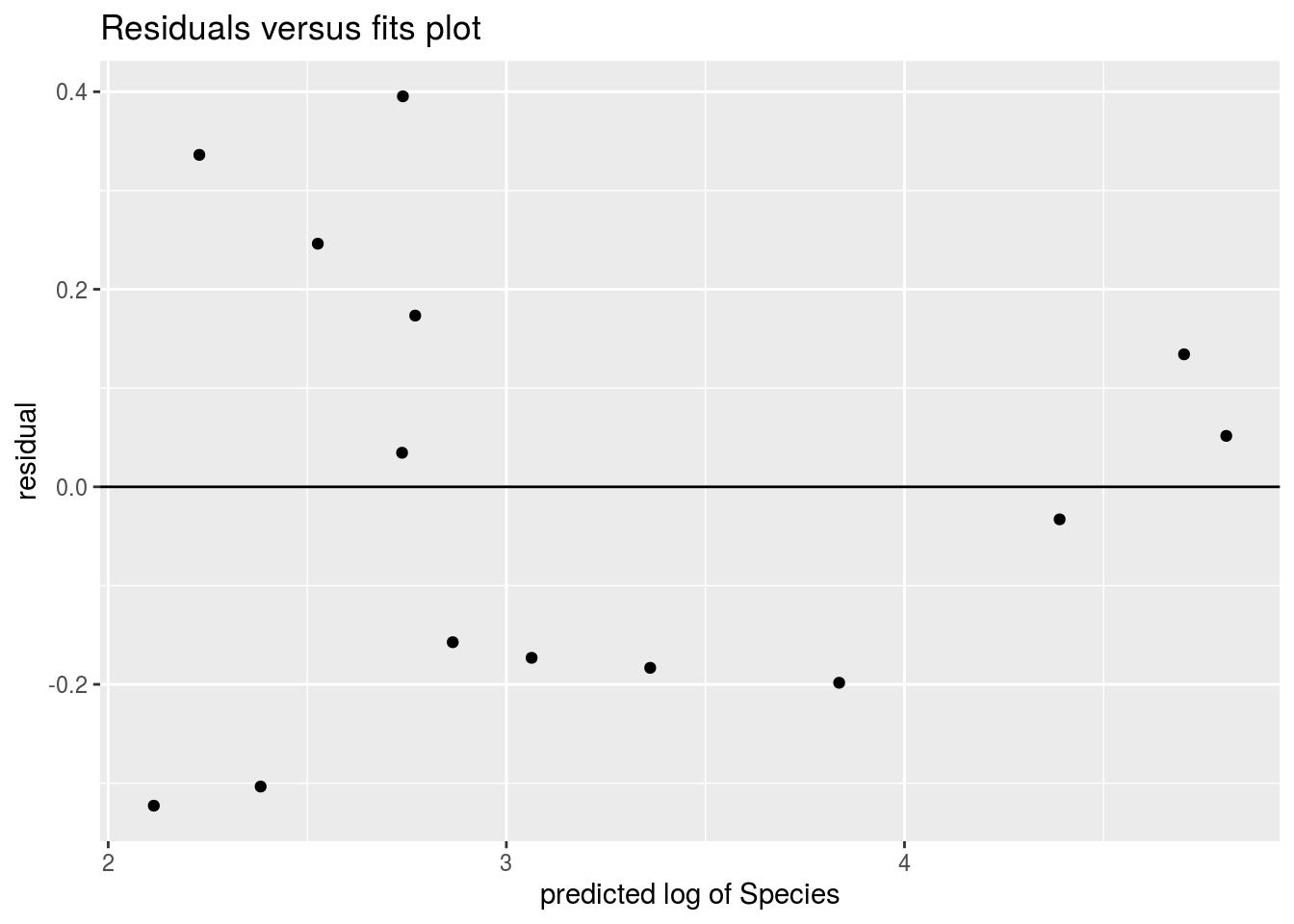library(tidyverse)
library(Stat2Data)
data("SpeciesArea")

## Create a scatter plot of Area on the x-axis and Species on the y-axis

ggplot(SpeciesArea, aes(x = Area, y = Species)) +
geom_point() +
labs(title = "Scatter plot of Area vs Species")## Create a scatter plot of Area on the x-axis and log(Species) on the y-axis

ggplot(SpeciesArea, aes(x = Area, y = log(Species))) +
geom_point() +
labs(title = "Relationship between Area and Log of Species")## Create a scatter plot of log(Area) on the x-axis and log(Species) on the y-axis

ggplot(SpeciesArea, aes(x = log(Area), y = log(Species))) +
geom_point() +
labs(title = "Relationship between log (Area) and log(Species)")## Fit a simple linear regression of logSpecies ~ logArea

model <- lm(log(Species) ~ log(Area), data = SpeciesArea)

## Examine the residuals vs. fits plot for the model logArea ~ logSpecies

SpeciesArea %>%
mutate(y_hat = predict(model),
residual = log(Species) - y_hat) %>%
ggplot(aes(x = y_hat, y = residual)) +
geom_point() +
geom_hline(yintercept = 0) +
labs(title = "Residuals versus fits plot",
x = "predicted log of Species")